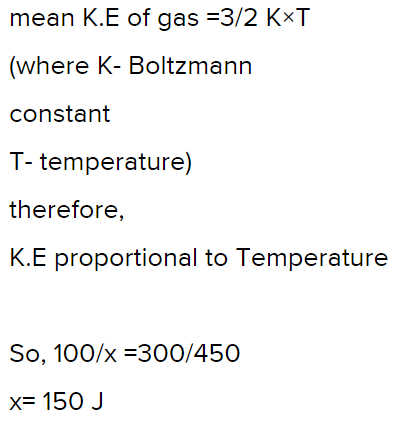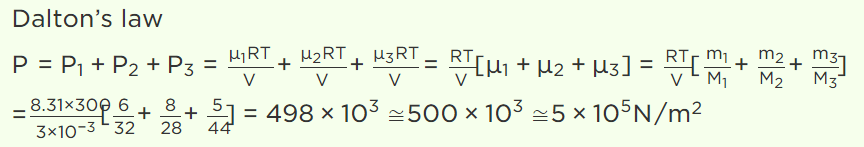## Kinetic Theory Questions and Answers Part-26

1. The mean kinetic energy of a gas at 300 K is 100 J. The mean energy of the gas at 450 K is equal to
a) 100 J
b) 3000 J
c) 450 J
d) 150 J

Explanation:2. The capacity of a vessel is 3 litres. It contains 6 gm oxygen, 8 gm nitrogen and 5 gm $CO_{2}$ mixture at 27°C. If R = 8.31 $J/mole\times kelvin$     , then the pressure in the vessel in $N\diagup m^{2}$  will be (approx.)
a) $5\times10^{5}$
b) $5\times10^{4}$
c) $10^{6}$
d) $10^{5}$

Explanation:3. In the absence of intermolecular force of attraction, the observed pressure P will be
a) P
b) < P
c) > P
d) Zero

Explanation: In the absence of intermolecular force of attraction, the observed pressure P will be > P

4. Two ideal gases at absolute temperature $T_{1}$  and $T_{2}$  are mixed. There is no loss of energy. The masses of the molecules are $m_{1}$  and $m_{2}$  and the number of molecules in the gases are $n_{1}$  and $n_{2}$  respectively. The temperature of mixture will be
a) $\frac{T_{1} + T_{2} }{2}energy$
b) $\frac{T_{1} + T_{2} }{n_{1} n_{2}}$
c) $\frac{n_{1}T_{1} +n_{2} T_{2} }{n_{1}+ n_{2}}$
d) $\left(T_{1} + T_{2}\right)$

Explanation: $\frac{n_{1}T_{1} +n_{2} T_{2} }{n_{1}+ n_{2}}$

5. The molecules of an ideal gas at a certain temperature have
a) Only potential energy
b) Only kinetic energy
c) Potential and kinetic energy both
d) None of the above

Explanation: The molecules of an ideal gas at a certain temperature have only kinetic energy

6. Mean kinetic energy per degree of freedom of gas molecules is
a) $\frac{3}{2}kT$
b) kT
c) $\frac{1}{2}kT$
d) $\frac{3}{2}RT$

Explanation: Mean kinetic energy per degree of freedom of gas molecules is $\frac{1}{2}kT$

7. The temperature at which the average translational kinetic energy of a molecule is equal to the energy gained by an electron in accelerating from rest through a potential difference of 1 volt is
a) $4.6\times 10^{3}K$
b) $11.6\times 10^{3}K$
c) $23.2\times 10^{3}K$
d) $7.7\times 10^{3}K$

Explanation:8. The kinetic energy of one gm-mole of a gas at normal temperature and pressure is (R = 8.31 J/Mole-K)
a) $0.56\times 10^{4}J$
b) $1.3\times 10^{2}J$
c) $2.7\times 10^{2}J$
d) $3.4\times 10^{3}J$

Explanation:9. The average kinetic energy of hydrogen molecules at 300 K is E. At the same temperature, the average kinetic energy of oxygen molecules will be
a) E/4
b) E/16
c) E
d) 4 E

10. The average translational kinetic energy of a hydrogen gas molecules at NTP will be [Boltzmann’s constant $K_{B}=1.38\times 10^{-23}J\diagup K$     ]
a) $0.186\times 10^{-20}Joule$
b) $0.372\times 10^{-20}Joule$
c) $0.56\times 10^{-20}Joule$
d) $5.6\times 10^{-20}Joule$